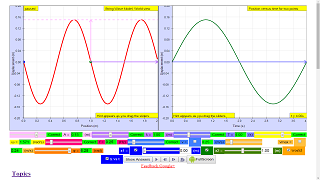Translations

Code Language Translator RunSoftware Requirements

SoftwareRequirements

 Android iOS Windows MacOS with best with Chrome Chrome Chrome Chrome support full-screen? Yes. Chrome/Opera No. Firefox/ Samsung Internet Not yet Yes Yes cannot work on some mobile browser that don't understand JavaScript such as..... cannot work on Internet Explorer 9 and below

Credits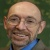Andrew Duffy; lookang; tina

end faq

http://iwant2study.org/lookangejss/04waves_12generalwaves/ejss_model_Wave_representations_v5/Wave_representations_v5_Simulation.xhtml

Apps

Description

Introduction
Wave is an oscillation accompanied by a transfer of energy that travels through medium (space or mass)
Waves consist, instead, of oscillations or vibrations (of a physical quantity), around almost fixed locations
This simulation is on transverse wave where disturbance creates oscillations that are perpendicular to the propagation of energy transfer.
The equation for wave is A sin ( wt +kx + ϕ)
where
A is the maximum amplitude of the wave, maximum distance from the highest point of the disturbance in the medium (the crest) to the equilibrium point during one wave cycle.
w is is the angular frequency
t is time
k is is the wavenumber
x is the position
ϕ is the is the phase constant

concepts illustrated in the simulations include
T is the time for one complete cycle of an oscillation of a wave
f is the number of periods per unit time (per second) and is related by T = 1/f
λ is the wavelength
v is the velocity of the wave travelling and is related by v= f λ
vtmax is the maximum transverse velocity of the wave particle that occurs at the displacement d =0.

Examples

EJSS wave representation model

Equation used to model the wave is $$y = A sin ( \omega t - k x )$$

Amplitude A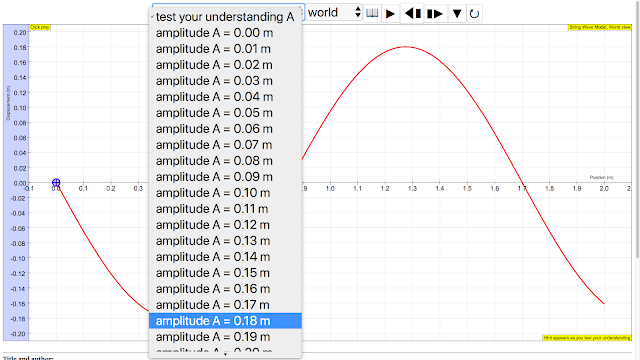amplitude of wave = 0.18 m, maximum displacement from equilibrium positionhttp://iwant2study.org/ospsg/index.php/112Direct Link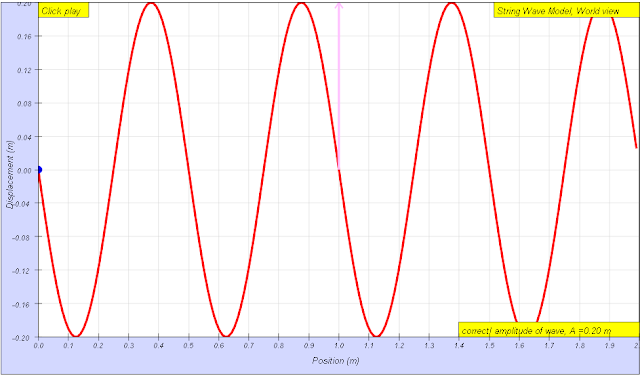amplitude of wave = 0.20 m, maximum displacement from equilibrium position run: Link1, Link2 download: Link1,source:  author: Andrew Duffy, lookang, tina author EJS: Francisco Esquembre

wavelength λwavelength of wave = 1.70 m The wavelength λ  wavelength is the distance between two sequential crests or troughs (or other equivalent points)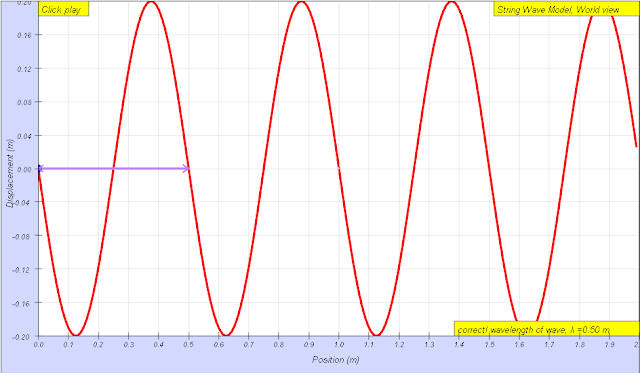wavelength of wave = 0.50 m The wavelength λ  wavelength is the distance between two sequential crests or troughs (or other equivalent points) run: Link1, Link2 download: Link1, Link2source:  author: Andrew Duffy, lookang, tina author EJS: Francisco Esquembre

Period Tperiod of wave = 3.00 s, The period T is the time for one complete cycle of an oscillation of a wavehttp://iwant2study.org/ospsg/index.php/112Direct Linkperiod of wave = 3.00 s, The period T is the time for one complete cycle of an oscillation of a wave run: Link1, Link2 download: Link1, Link2source:  author: Andrew Duffy, lookang, tina author EJS: Francisco Esquembre

angular frequency fThe frequency f = 1/T  is the number of periods per unit time (per second) and is typically measured in hertzhttp://iwant2study.org/ospsg/index.php/112Direct LinkThe frequency f = 1/T is the number of periods per unit time (per second) and is typically measured in hertz run: Link1, Link2 download: Link1, Link2source:  author: Andrew Duffy, lookang, tina author EJS: Francisco Esquembre

angular velocity of wave ωangular frequencyω  = 2.09 s, The angular frequencyω represents the frequency in radians per second. run: Link1, Link2 download: Link1, Link2source:  author: Andrew Duffy, lookang, tina author EJS: Francisco Esquembre

phase difference ϕthe phase of a vibration (that is, its position within the vibration cycle) ϕ measured in radians taking ratio "ϕ/(2π)= Δt/T",  ϕ = 2π(2.5-0.75)/3 =3.7 rad approximately

wave speed v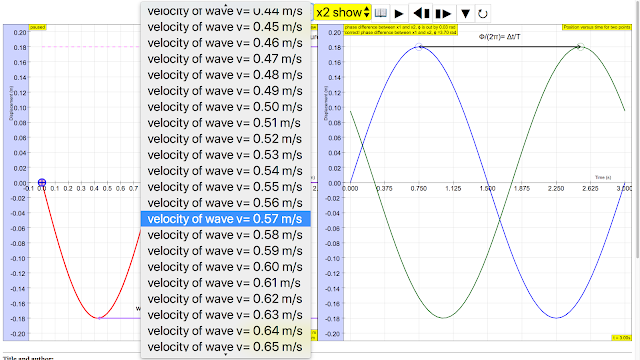sinusoidal waveform traveling at constant speed v is given by v = fsinusoidal waveform traveling at constant speed v is given by v = f λ run: Link1, Link2 download: Link1, Link2source:  author: Andrew Duffy, lookang, tina author EJS: Francisco Esquembre

maximum transverse speed vmaxThe maximum transverse velocity is vmax = Aω and it occurs when the particle on the wave travels passes through the equilibrium positionhttp://iwant2study.org/ospsg/index.php/112Direct LinkThe maximum transverse velocity is vmax = Aω and it occurs when the particle on the wave travels passes through the equilibrium position run: Link1, Link2 download: Link1, Link2source:  author: Andrew Duffy, lookang, tina author EJS: Francisco Esquembre

reference:

http://weelookang.blogspot.sg/2011/04/ejs-open-source-wave-representations.html

Versions

1. http://weelookang.blogspot.sg/2015/08/ejss-wave-representation-model.html Blog post of JavaScript version of Wave Representations by Andrew Duffy and Loo Kang Wee
2. http://weelookang.blogspot.sg/2011/04/ejs-open-source-wave-representations.html Blog post of Java version of Wave Representations by Andrew Duffy and Loo Kang Wee
3. http://iwant2study.org/lookangejss/04waves_12generalwaves/ejs/ejs_model_Wave_representations_v5.jar Java version of Wave Representations by Andrew Duffy and Loo Kang Wee

Other Resources

1. http://physics.bu.edu/~duffy/HTML5/wave_movie_and_graph.htmlby Andrew Duffy
2. http://www.phy.ntnu.edu.tw/ntnujava/index.php?topic=2405.0 One dimensional moving wave y(x,t)=A sin(k*x-w*t) by  Fu-Kwun Hwang

end faq